# To Determine The Internal Resistance Of A Given Primary Cell Using A Potentiometer

## Aim

To determine the internal resistance of a given primary cell using a potentiometer.

## Materials Required

1. 1 galvanometer
2. A battery
3. 1 potentiometer
4. 1 rheostat of low resistance
5. A fractional resistance box
6. 1 ammeter
7. 2 numbers keys (one-way)
8. Connecting wires
9. A jockey
10. 1 high resistance box
11. Sandpaper
12. 1 Leclanche cell
13. 1 set square
14. 1 voltmeter

## Theory

The potentiometer is a device used to measure the internal resistance of a cell and is used to compare the e.m.f. of two cells and potential difference across a resistor. The relation between potential difference, emf, and internal resistance of a cell is given by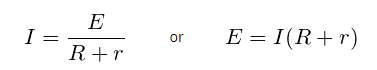## Hence V=IR = E-Ir

This indicates the value of V is less than E by an amount equal to the fall of potential inside the cell due to its internal resistance.

From the above equation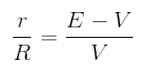## The internal resistance of the cell is given by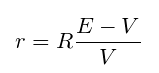##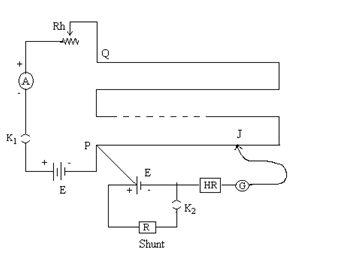Here the internal resistance of the cell is given by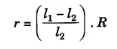Where l1 and l2 are balancing lengths without shunt or with the shunt. R is the shunt resistance in parallel with the given cell.

## Procedure

1. The connections should be according to the diagram shown above.
2. Using sandpaper clean the ends of the connecting wires and make sure that the connections are tight.
3. The plugs in the resistance box should be tight.
4. The e.m.f of the cell and battery is more than that of the cell. If it is not then the null point won’t be obtained.
5. Rheostat resistance can be made minimum by taking maximum current from the battery.
6. To check if the circuit connections are correct, the galvanometer deflections should be in the opposite direction. This is done by inserting the key K1 and making note of ammeter reading.
7. To obtain the null point on the fourth wire, the rheostat should be adjusted without inserting the key K2.
8. Take the small resistance between 1-5 ohm from resistance box R connected in parallel with the cell.
9. Slide the jockey and obtain the null point

## Observations

The least count of voltmeter = ………

Range of voltmeters = ……..

E.M.F of cell = ………

E.M.F of battery = ……

Table for lengths

 Sl.no Corrected ammeter reading (A) Balance point when E1 (Leclanche cell) in the circuit l1 (cm) Balance point when E2 (Daniel cell) in the circuit l2 (cm) (E1/E2) = (l1/l2)

## Calculations

1. For each set of observation find mean and l2
2. Calculate the value of r for each set.
3. Take the mean of values of r.

## Result

The internal resistance of the given cell is found to be………

## Precautions

1. The e.m.f of the cell should be lesser than the battery.
2. Have an eye to make sure that the ammeter reading remains constant at least for a single set of readings.
3. Ensure the current is passed only while obtaining the null point.
4. The rheostat should be placed
5. During the experiment, the cell should not be disturbed.
6. There shouldn’t be rubbing of jockey against potentiometer wire.

## Viva Questions

Q1. What does the e.m.f of a cell mean?

Ans: e.m.f stands for electromotive force. E.M.F a cell means the potential difference across the terminals of the cell.

Q2. What is a potentiometer?

Ans: Potentiometer is defined as an instrument used for measuring the potential difference of a cell.

Q3. Why is it called potentiometer?

Ans: It is called a potentiometer because it measures the potential difference between any two points in an electric circuit.

Q4. What is the principle of a potentiometer?

Ans: The principle behind the potentiometer is that for a constant current, the fall of potential in a wire is directly proportional to the length of the wire.

Q5. What is the potential gradient?

Ans: Potential gradient is defined as the potential difference per unit length of wire